•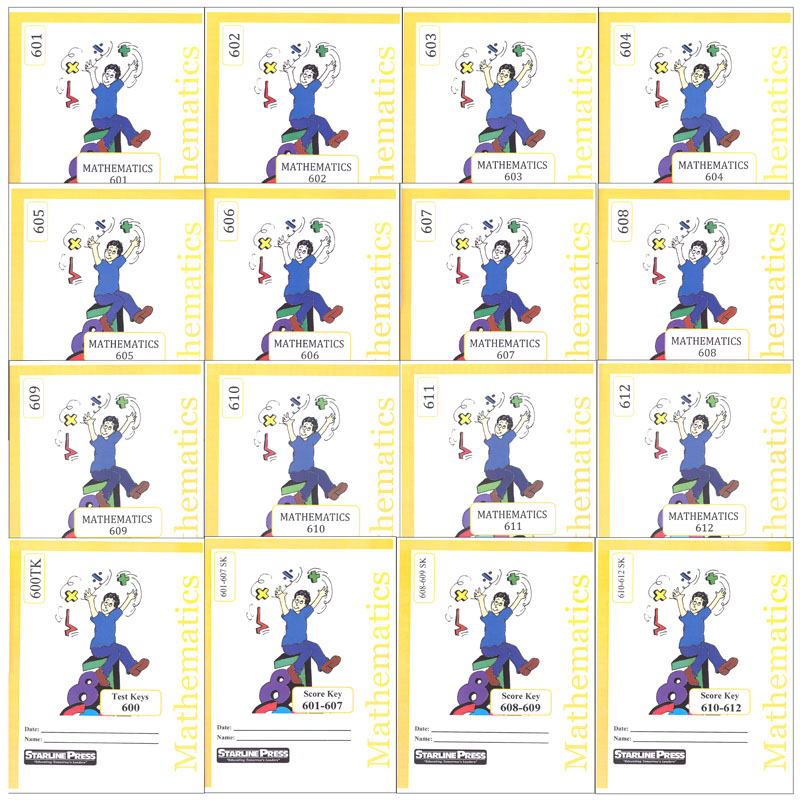•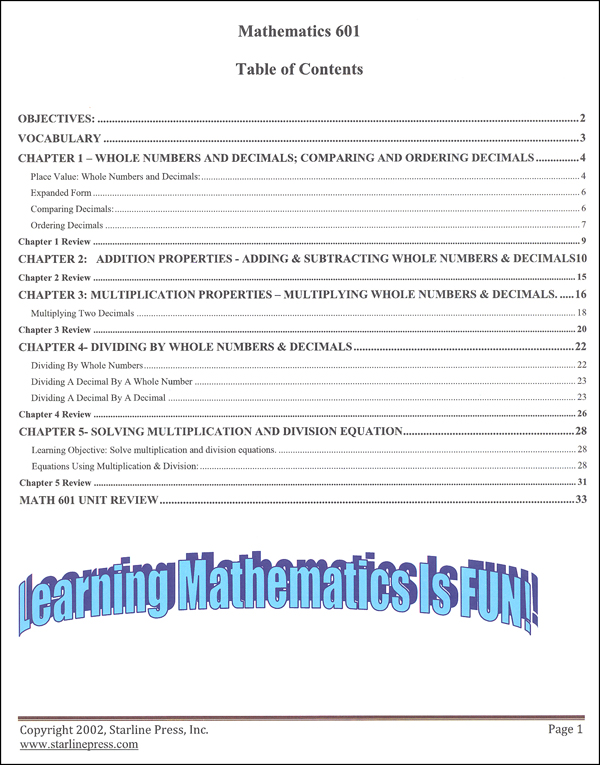•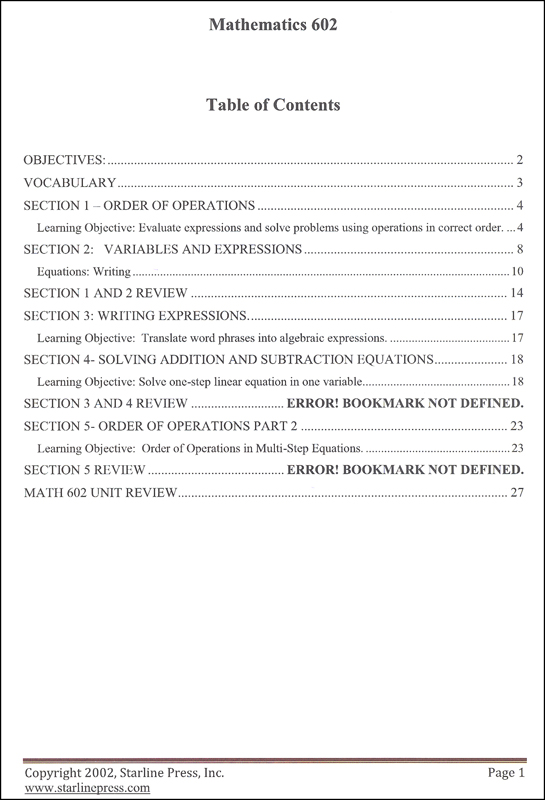•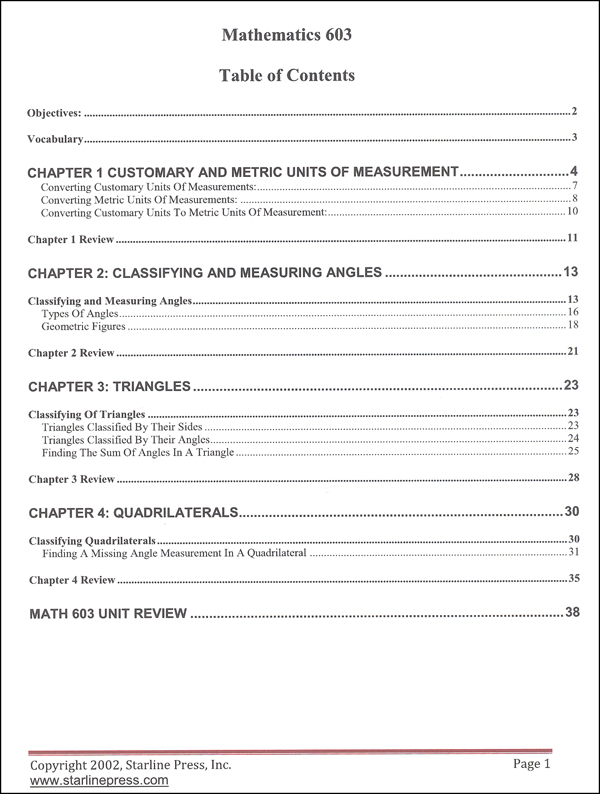•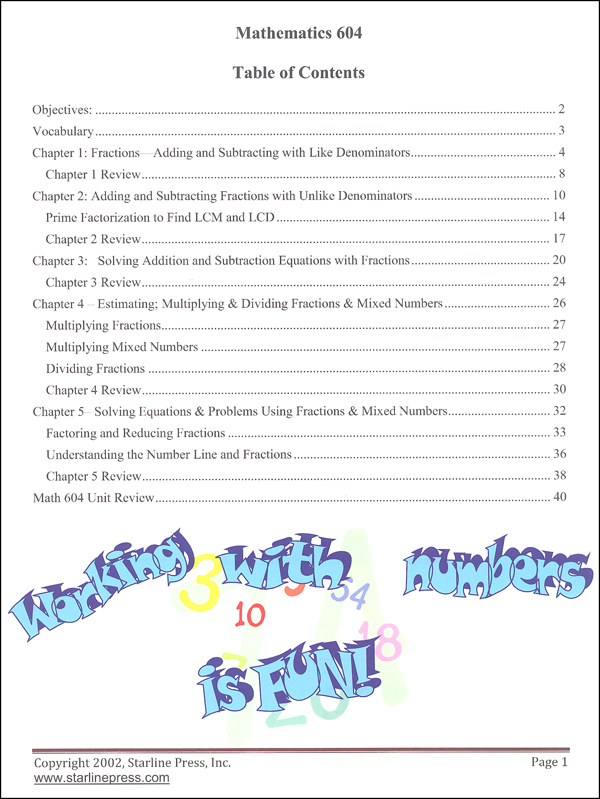•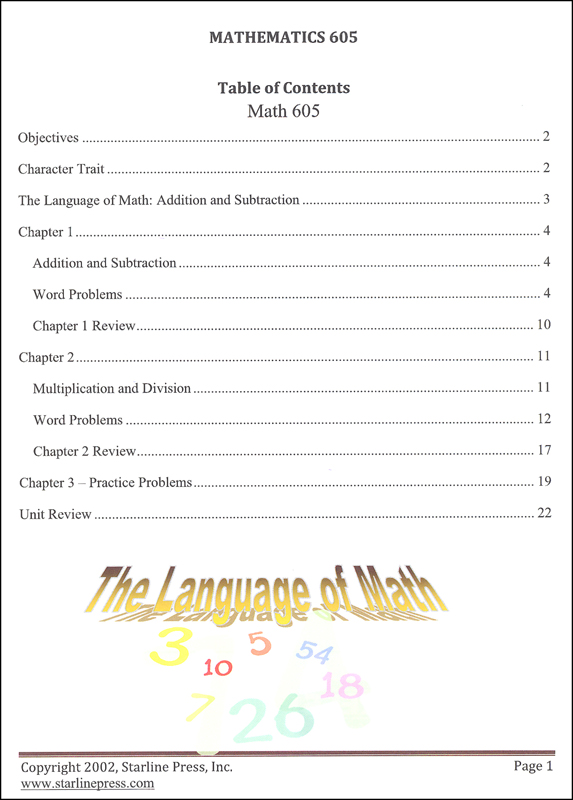•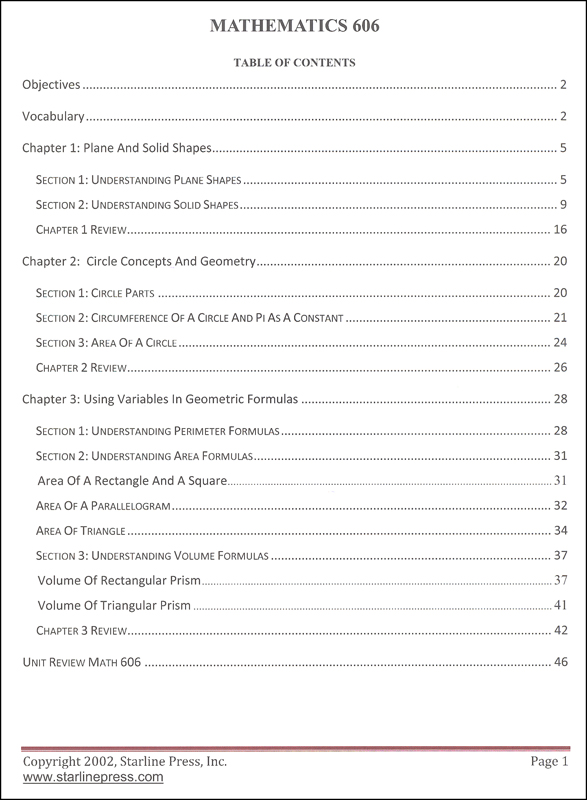•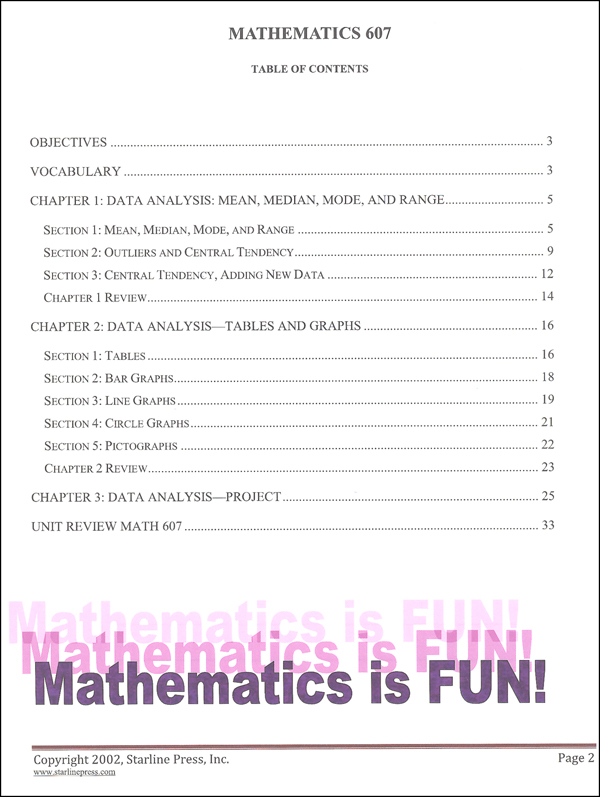•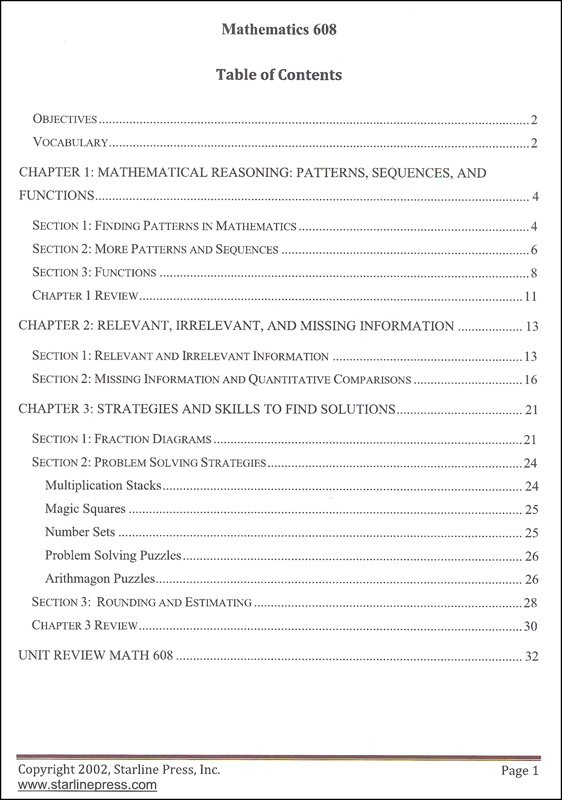•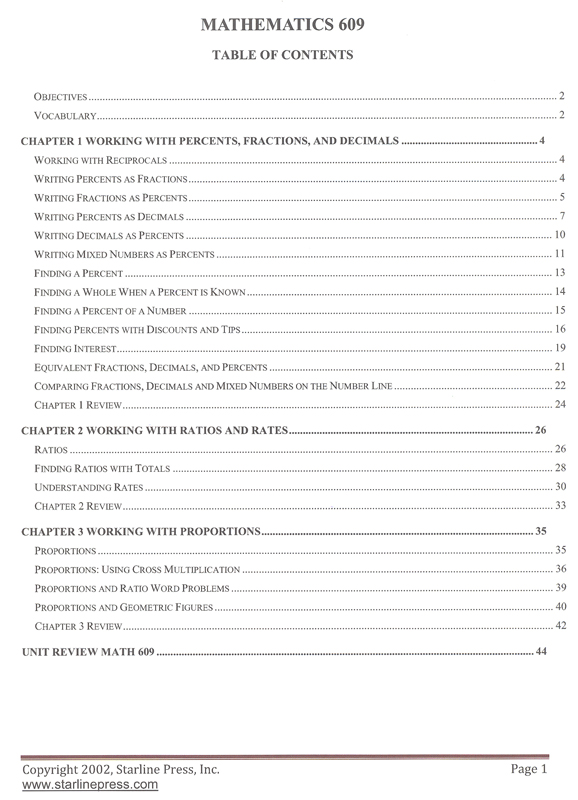•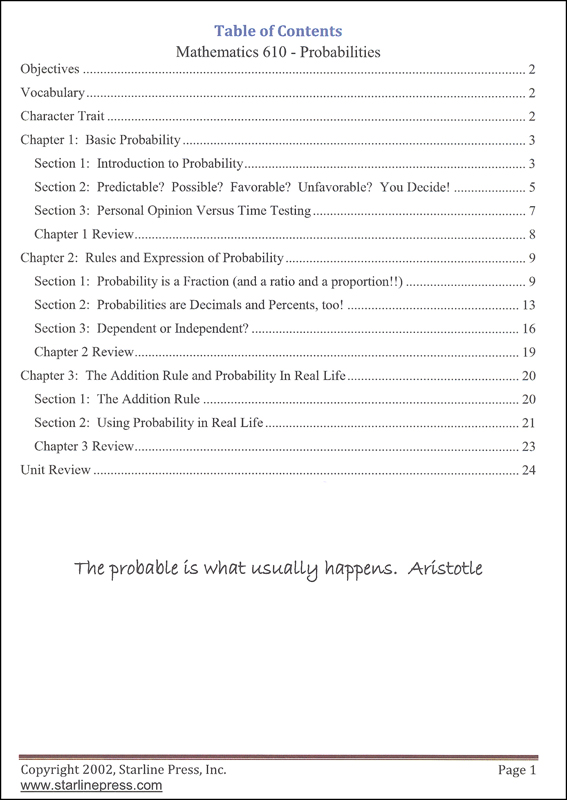•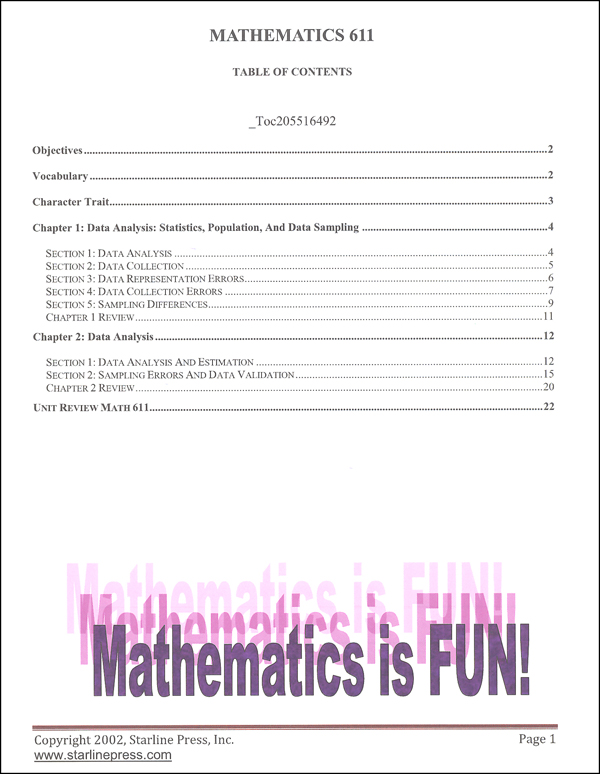•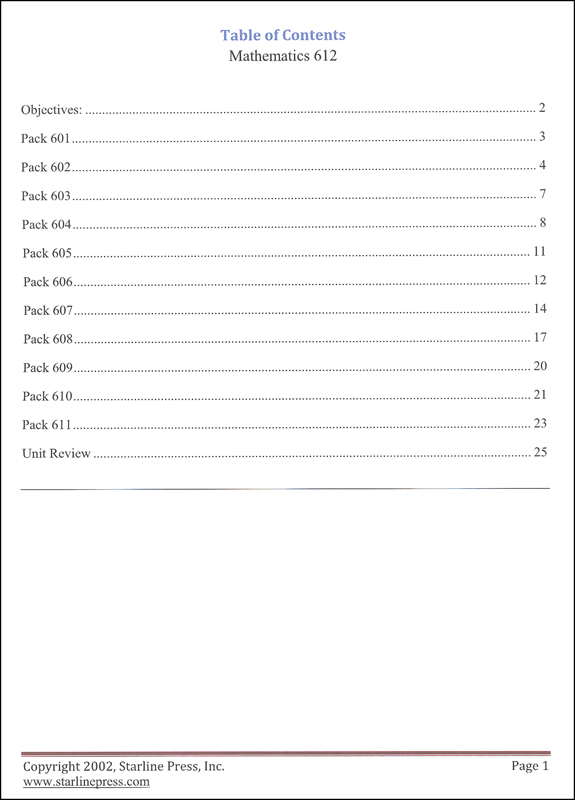•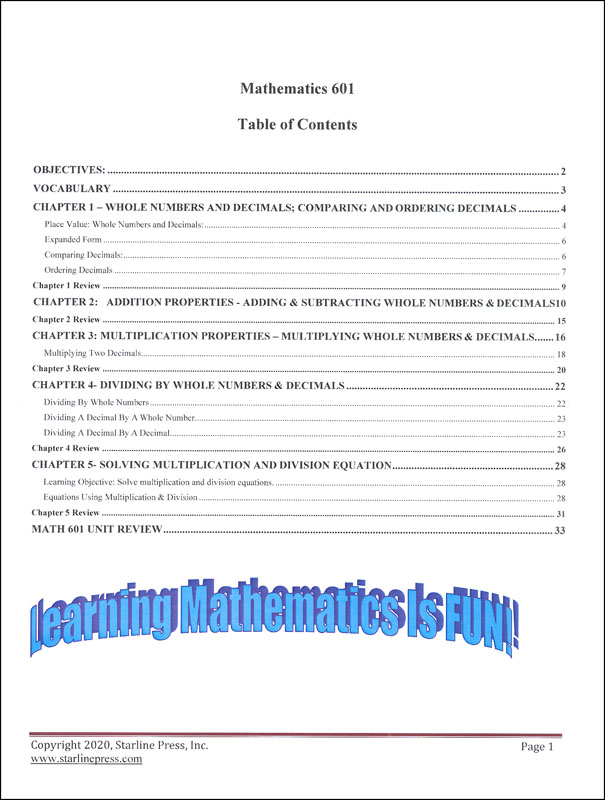•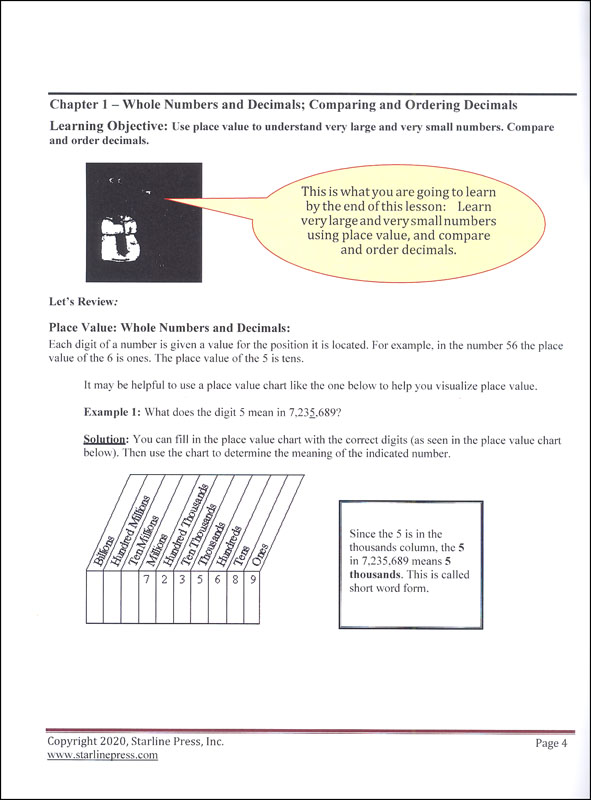••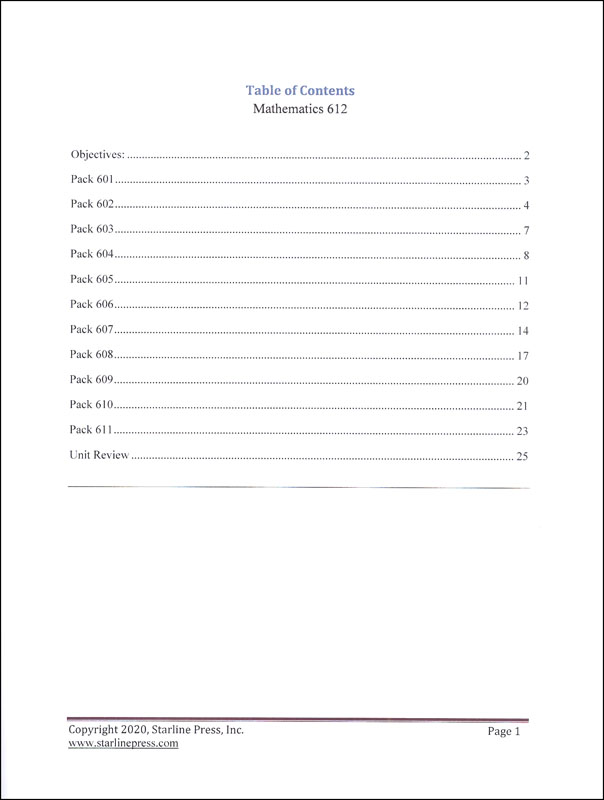•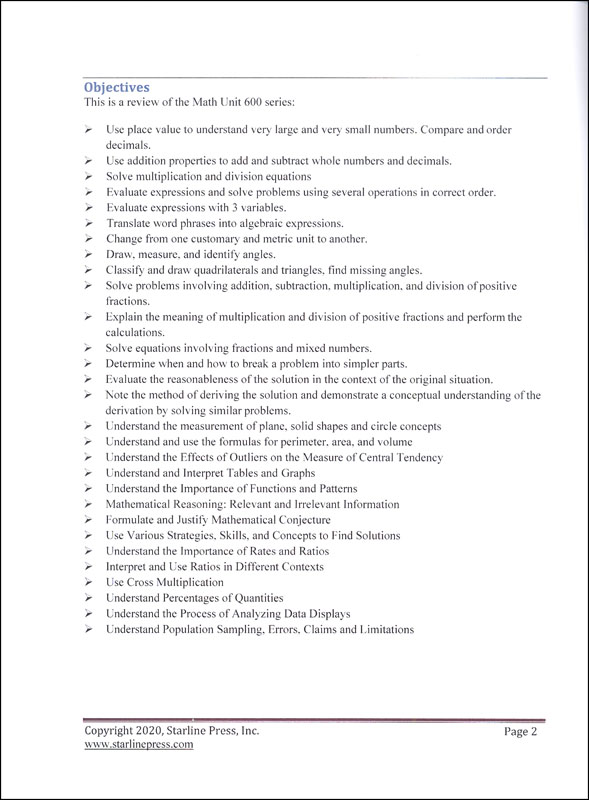•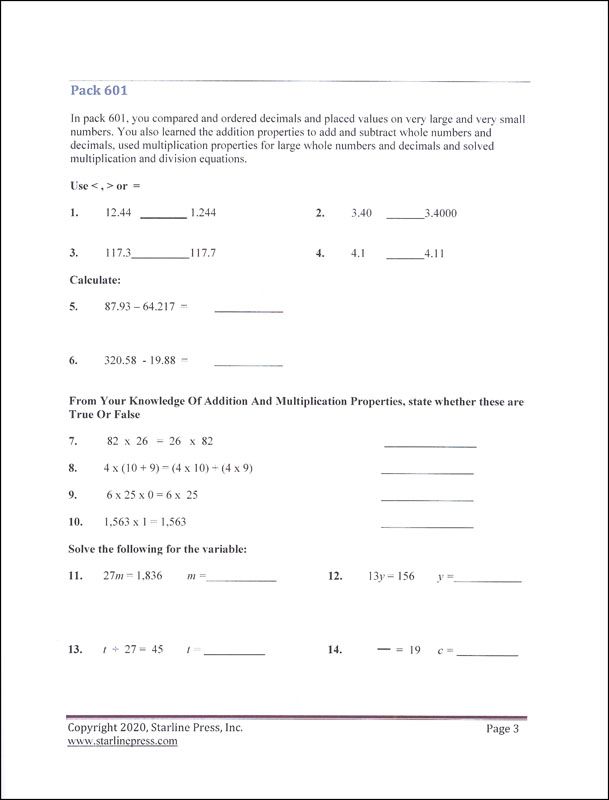•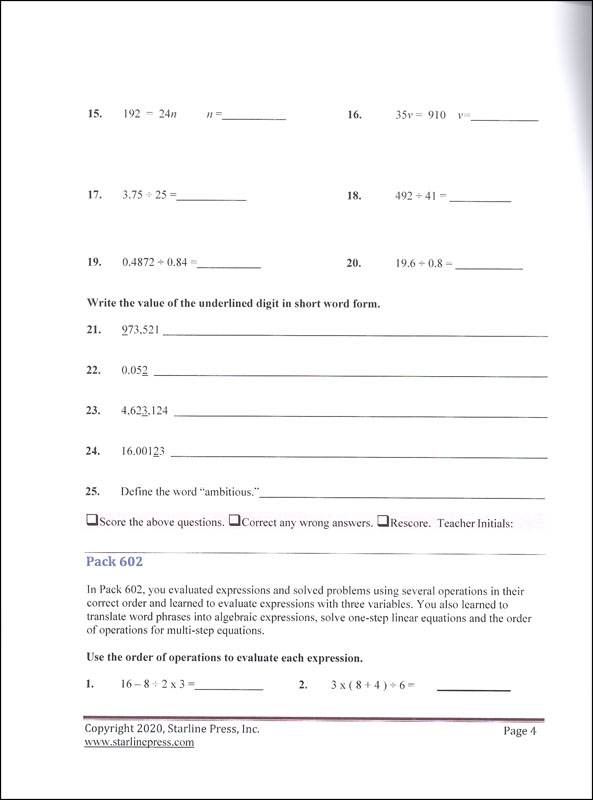# Math 6th Grade Set

# 051462

Our Price: \$199.95
Retail: \$218.71
Save: 8.58% (\$18.76)
In Stock.
Qty:
Qty:

 Item #: 051462 6

#### Product Description:

Students will multiply and divide using whole numbers and decimals. They solve equations and problems using fractions and mixed numbers. Study of geometric formulas, data analysis and mathematical reasoning is included. Students will work with percent, ratios, and proportions. Probability and statistics is studied using data analysis and estimation. Geometry, symmetry and spatial relationships are taught.

#### Publisher Description:

Sixth grade students multiply and divide using whole numbers and decimals. They solve equations and problems using fractions and mixed numbers. They study geometric formulas, data analysis, and mathematical reasoning. The sixth grade student works with percents, ratios, and proportions. They study probability and statistics using date analysis and estimation. Sixth grade students study geometry, symmetry, transformations, and spatial relationships.

Category Description for Starline Press Math:

Starline Press Math is a character-based, individualized and independent learning curriculum. Aligned to pre-Common Core California state standards (please see publisher website for CA state standards correlation), each grade level provides self- directed instruction with minimal teacher supervision. Grades 3-8 complete twelve units (booklets) per year, with a suggested 3 week completion time frame. Grades 9-10 complete 6 booklets per semester /12 per year. Each grade level includes vocabulary, fill in the blank questions, practice problems, chapter reviews, and unit tests. Consumable worktexts are colorfully illustrated soft covers, although newer printings are more like booklets and do not have the perfect binding and glossy covers. Available only in complete sets which include answer and test keys. ~ Deanne

Category Description for COMPREHENSIVE PROGRAMS - ALL GRADES:

Primary Subject
Mathematics
6
Format
Softcover Book Set
Brand Name
Starline Press
Weight
3.4 (lbs.)
Dimensions
11.0" x 8.75" x 2.0"